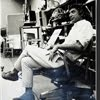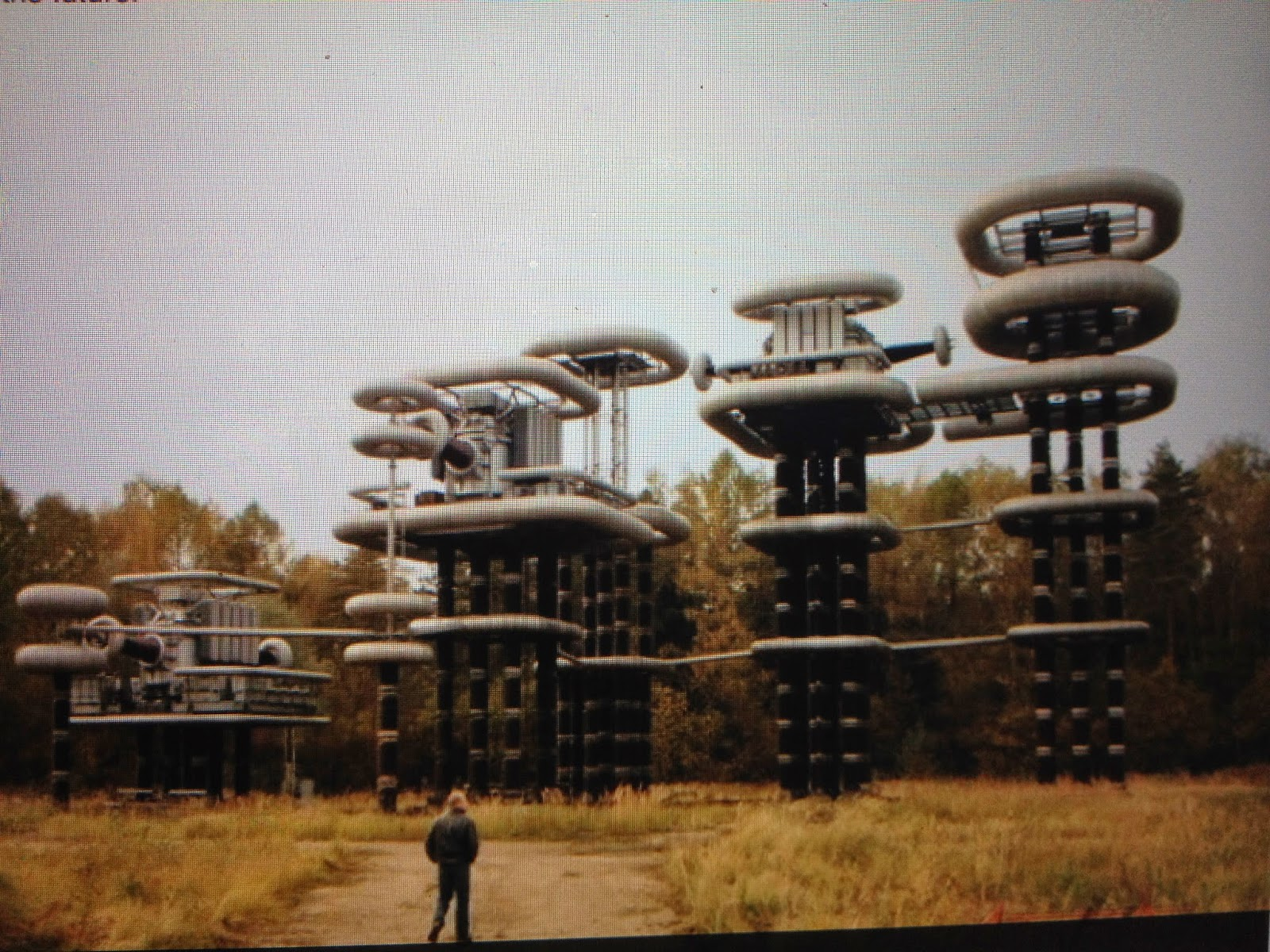## Eugene Ray SDSU 1970'sEugene Ray 1970's. photo credit: Tom Davis

## Thursday, July 10, 2014

### TRANSCENDENT MANIFESTS / biotronic prototype structures

+TESLA BIOTRONIC MACHINE+
>constructed by the "russian electrical engineering institute"<
>coupled with the prototype russian pyramid structures I<
+RUSSIAN PYRAMIDS+
>featured in the blog previously reflect the profound<
>perspective of russian biotronic energy research<
^^^^^^^^^^^^^^^^^^^^^^^^^^^^^^^^
+TRANSCONTINENTAL WIRELESS+
+>>ENERGY TRANSMISSIONS<<+
>the great vision of nicola tesla is the goal<
>of the russian piekhanov brothers who<
>want to rebuild his biotronic towers<
^^^^^^^^^^^^^^^^^^^^^^
+PERSPECTIVE+
>sdsu alum in my 70s classes will recall the book<
>"psychic discoveries behind the iron curtain" as<
+ENERGY RESEARCH)+
>a reference to advanced profound research in<
>russia relating to the use of natural biotronics<
^^^^^^^^^^^^^^^^^^^^^^^^^^^^
>parallel to this research was the research and<
>book by my friend patrick flanigan phd on<
+"PYRAMID POWER"+
>flanigan a child prodigy was featured in<
>life magazine ref  his device for the deaf<
^^^^^^^^^^^^^^^^^^^^^^^^^
>introduction to my russian pyramid image refs<
^^^^^^^^^^^^^^^^^^^^^^^^^
>first image page features my favorite prototype<
^^^^^^^^^^^^^^^^^^^^^^^^^^
>i am grateful to "google" for these three pages<
>of my blog images that have tangental ref. to<
+/ MY PYRAMID BLOGS)+
>my previous blogs about russian pyramids<
^^^^^^^^^^^^^^^^^^^^^^^^^^^^
>energy transmission from pyramids, both<
+(INFRARED PHOTO OF+
>full size pyramids & model pyramids<
^^^^^^^^^^^^^^^^^^^^^^^^
>dr. alexander golod has built and tested over<
>twenty full size pyramids as we built and<
+(OUR TESTS IN THE 70S+
+ALL WERE POSITIVE)+
>tested model pyramids in the early 70s<
^^^^^^^^^^^^^^^^^^^^^^^^>internet available photographs from www.esosedi.ru<
>document the tesla inspired towers near moscow<
+(BIOTRONIC ENERGY+
+RESEARCH TOWERS)+
>relating to the plans of the pikehanov brothers<
>addressing the worlds serious energy needs<
^^^^^^^^^^^^^^^^^^^^^^^^^^^>i am reminded of charles gayarre growing up on<
>the de bore-foucher plantations where the sugar<
+(TULANE UNIVERSITY+
+RESEARCH LOCUS)+
>processing >inventions< saved the plantations<
^^^^^^^^^^^^^^^^^^^^^^^^^^^
>i am reminded that extraterrestrial knowledge<
>is well documented in the construction of<
+BOOK "GODS MACHINES")+
>ancient structures using biotronic energy<
^^^^^^^^^^^^^^^^^^^^^^^^
>"the universal spheroid" openable double teflon<
>skin garden prototype residence reflects energy<
+UNIVERSAL SPHEROID)+
>research regarding biotronics in sphere volume<
^^^^^^^^^^^^^^^^^^^^^^^^^^^^
>for thirty years the exhibitions i mounted<
>documented many prototypes utilizing<
+(NATURE IS OUR+
+BEST GUIDE)+
>electromagnetic natural energy design<
^^^^^^^^^^^^^^^^^^^^^^^^^
>in the case of this residential prototype<
>biotronic energy is utilized from both<
+BIO-STRUCTURE)+
>pyramid and spheroid volumes<
^^^^^^^^^^^^^^^^^^^^^
+OIL INTERESTS SHOULD NOT+
>(electromagnetic and solar<
>energies are natural / free)<
+DIRECT OUR ENERGY USE+ !
^^^^^^^^^^^^^^^^^^^^^^
>is this why i was once accused<
+OF BEING A COMMUNIST+ ?
^^^^^^^^^^^^^^^^^^^^^
eugene ray, mfa, architect
professor emeritus, sdsu